# Module 3: Reactive Chemistry | Beginner’s Guide To Year 11 Chemistry

Struggling with Year 11 Reactive Chemistry? In this Guide, we help you become proactive rather than reactive by guiding you through this subject.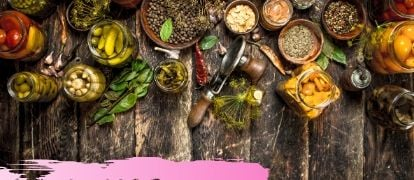In this article, we introduce reactive chemistry – how chemical reactions will take place. You’ve already seen chemical reactions in the previous module, but this information will help you to predict what chemical reaction will occur.

• Chemical Reactions
• Modelling Chemical Reactions
• Classifying Chemical Reactions
• Aboriginal and Torres Strait Islander People’s Detoxification of Food
• Predicting Reactions of Metals
• The Metal Activity Series
• Types of Reactions and Trends in Reactivity
• Introduction to REDOX Chemistry
• Galvanic Cells
• Rates of Reaction
• Collision Theory and Activation Energy
• Factors Affecting Rates of Reaction

## Chemical Reactions

A chemical reaction involves the rearrangement of atoms through breaking of chemical bonds, and the formation of new chemical bonds.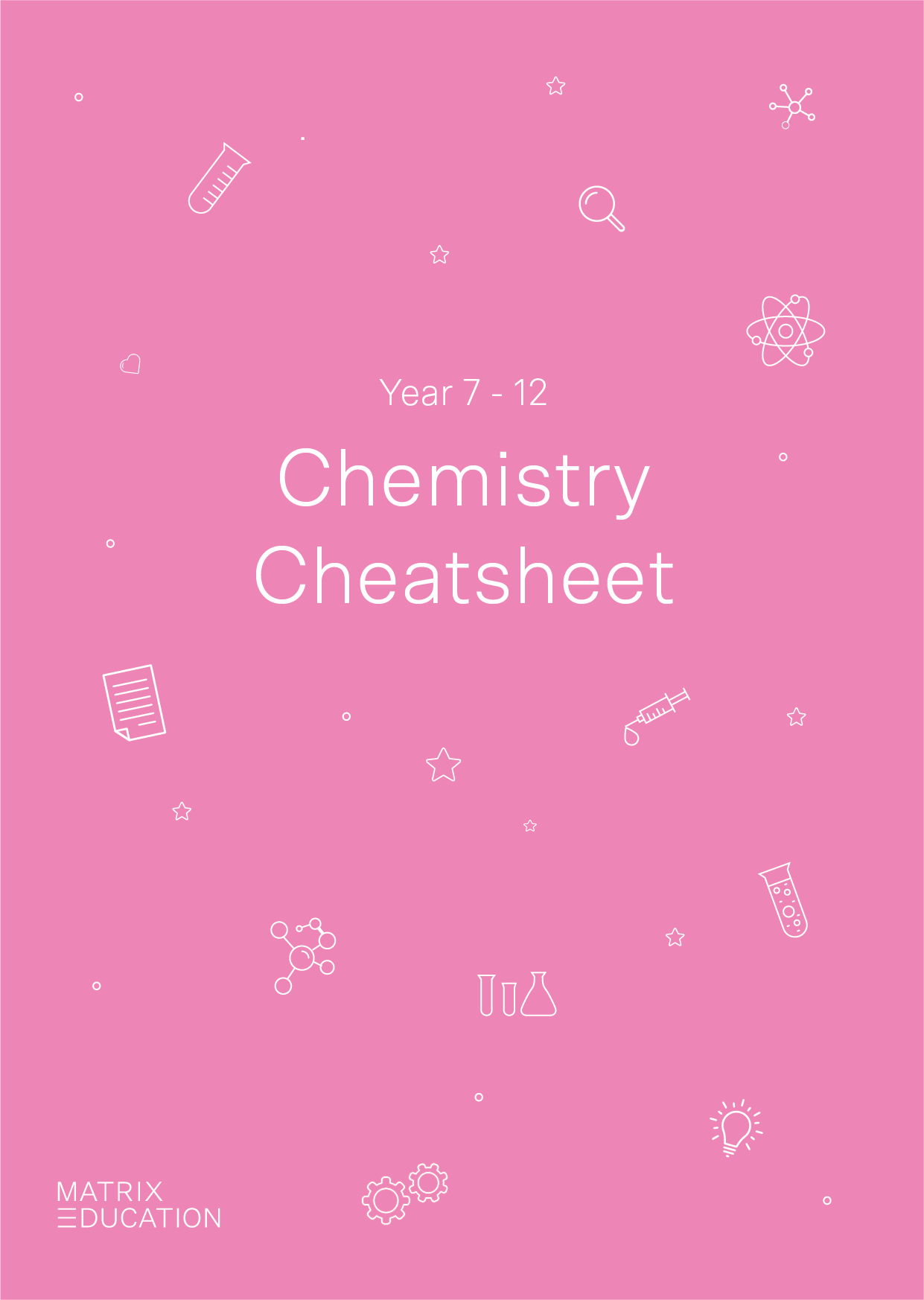A free pocket-sized cheatsheet, so you're always prepared.

Chemical reactions will often absorb or release energy from the surrounding environment.

If you can model a chemical reaction, this will allow you to understand the process that is occurring on a microscopic level.

### Modelling Chemical Reactions

In a chemical reaction, atoms are rearranged to form new substances.

The total number of each type of atom on each side of the chemical reaction (reactants and products) remains the same. We can represent this with a model.

A model is representation of an idea, object, process or system that is used to describe and explain phenomena.

We can use models to represent chemical reactions – these can be images, animations, videos, 3D kits or objects.

In the model below, we will use different coloured circles to represent the reaction of methane with oxygen to form carbon dioxide and water.

$$CH_{4(g)} + 2O_{2(g)} ⟶ CO_{2(g)} + 2H_2O_{(l)}$$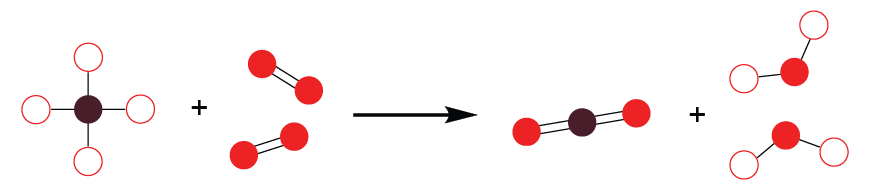In this model, the maroon circle represents carbon atoms, red unfilled circles represent hydrogen atoms and red filled circles represent oxygen atoms.

Importantly, this model shows the rearrangement of chemical bonds and that the equation is balanced (conservation of atoms).

### Classifying Chemical Reactions

In the chemistry described in the HSC you will encounter six common types of reaction.

You should be able to write the reactants and products for any of these reactions when provided with a small amount of information.

For example, you may be told the type of reaction and only one of the reactants.

These types are the 6 types of reactions:

1. Synthesis: The formation of a more complex substance from less complex substances.

Example: $$2H_{2(g)} + O_{2(g)}⟶ 2H_{2}O_{(l)}$$

2. Decomposition: The breakdown of a more complex substance to form less complex substances.

Example: $$CuCO_{3(s)} ⟶ CO_{2(g)} + CuO_{(s)}$$

3. Combustion: The reaction of a substance with oxygen to produce an oxide.

Example: $$CH_{4(g)} + 2O_{2(g)} ⟶ CO_{2(g)} + 2H_2O_{(l)}$$

4. Precipitation: The reaction of two aqueous substances to form an insoluble solid.

Example: $$Pb(NO_3)_{2(aq)} + 2KI_{(aq)} ⟶ PbI_{2(s)} + 2KNO_{3(aq)}$$

5. Neutralisation (Acid/Base Reaction): An acid and a base react to produce salt and water.

Example: $$NaOH_{aq} + HCl_{(aq)} ⟶ NaCl_{(aq)} + H_{2}O_{(l)}$$

6. Acid/Carbonate Reaction: An acid and a carbonate react to produce salt, water and carbon dioxide.

Example: $$Na_2CO_{3(aq)} +2HCl{(aq)} ⟶ 2NaCl{(aq)} + CO_{2(g)} + H_2 O_{(l)}$$

### Aboriginal and Torres Strait Islander Peoples Detoxification of Food

Many plants contain chemicals that are toxic to humans.

For example, Atropa belladonna (also called deadly nightshade) is a poisonous plant which contains toxins that can severely affect the nervous system.

However, some plants that contain toxins also contain parts that are nutritious and can be used as foods.

In order to use these plants as a food source, many indigenous peoples around the globe have developed methods to detoxify (remove toxins from) foods in order to consume them.

Some of these foods include:

• Yellow Walnut (Beilschmiedia bancroftii)
• Black Walnut (Endiandra palmerstonii)
• Black Pine Nut (Sundacarpus amara)
• Black Bean (Castanospermum austral)

Once these foods were collected, they were processed using one or more of the following methods:

1. Cooking: Heating decomposes toxic compounds.

2. Leaching: Water dissolves and removes toxic compounds.

3. Fermentation: Microorganisms decompose toxic compounds.

If you require more specific information, read this useful scientific paper.

## Predicting Reactions of Metals

Among the elements, metals are very common and also display very similar reactivity to one another. The following section will help you to predict the way metals will react with other substances.

### The Metal Activity Series

The metal activity series is an empirically derived list which arranges a variety of common metals from most reactive to least reactive.

A condensed version of this activity series is shown below, with metals grouped to reflect their reactivity.

A more reactive metal within a group appears at the top of the oval it is contained in.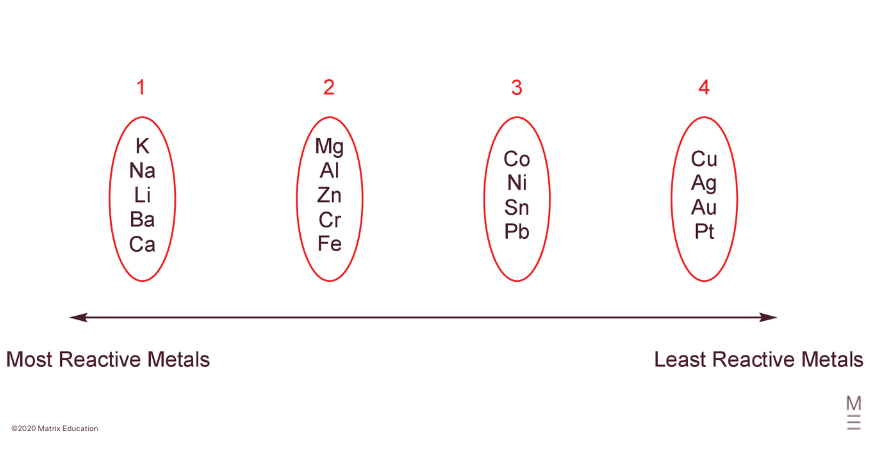In general, a more reactive metal will react more quickly with the same substance than a less reactive metal.

The metal activity series can be deduced from the type of reaction that a metal will have with a different substance.

In general, metals can be classed as:

• Very reactive (1)
• Reactive (2)
• Somewhat reactive (3)
• Unreactive (4) – NOTE: copper reacts slowly

These reactions are discussed in the section below.

### Types of Reaction

Depending on where a metal is located in the metal activity series, it may react with other substances.

A summary of these reaction types follows:

1. Metal and Water: A very reactive metal (1) will react with water to produce a metal hydroxide and hydrogen.

Example: $$2Na_{(s)} + 2H_2O_{(l)} ⟶ 2NaOH_{(aq)} + H_{2(g)}$$

2. Metal and Water: A reactive metal (2) will react with steam to produce a metal oxide and hydrogen.

Example: $$Zn_{(s)} +H_2O_{(g)} ⟶ ZnO_{(s)} + H_{2(g)}$$

3. Metal and Dilute Acid: A very reactive (1), reactive (2) or somewhat reactive (3) metal will react with dilute acid to produce a salt and hydrogen.

Example: $$Mg_{(s)} + 2HCl_{(aq)} ⟶ MgCl_{2(aq)} + H_{2(g)}$$

4. Metal and Oxygen: All reactive metals (1, 2, 3 and Cu) react with oxygen to produce metal oxides. Reactive (2) and somewhat reactive (3) metals require heat to do this.

Example: $$2Ca_{(s)} + O_{2(g)} ⟶ 2CaO{(s)}$$

5. Metals and Metal Ions: A more reactive metal will displace a less reactive metal from solution.

Example: $$Mg{(s)} + CuSO_{4(aq)} ⟶ Cu_{(s)} + MgSO_{4(aq)}$$

There are three important trends that correlate to the metal activity series:

1. The reactivity of metals increases with decreasing ionisation energy.
2. The reactivity of metals increases with increasing atomic radius.
3. The reactivity of metals increases with decreasing electronegativity.

Each of the above circumstances results in less energy being required for the metal to react, resulting in a more reactive metal.

### Introduction to REDOX Chemistry

Many important chemical processes, such as batteries, rely on the transfer of electrons from one substance to another.

When talking about electron transfer processes, it is important to know that:

• Oxidation Is Loss of electrons
• Reduction Is Gain of electrons

The mnemonic OIL RIG can be used to remember this relationship.

REDOX chemistry involves oxidation and reduction reactions.

To indicate the number of electrons that have been lost or gained from an ion, we use oxidation numbers.

You have encountered oxidation numbers before as the charge of an ion.

The pure form of an element such as $$Na_{(s)}$$ or $$Cl_{2(g)}$$ is assigned an oxidation number of 0.

The table below shows the oxidation number of ions formed from the period 2 elements.

 Ion Li+ Be2+ B3+ C4+ N3- O2- F- Ne Table:  Oxidation number of ions formed from the period 2 elements Oxidation number +1 +2 +3 +4 -3 -2 -1 0 Electrons lost 1 2 3 4 — — — — Electrons gained — — — — 3 2 1 —

### Galvanic Cells

Galvanic cells are a simple form of a battery – chemical potential energy is converted to electricity through the oxidation of one species and the reduction of another.

An example of a galvanic cell using nickel and copper is shown below.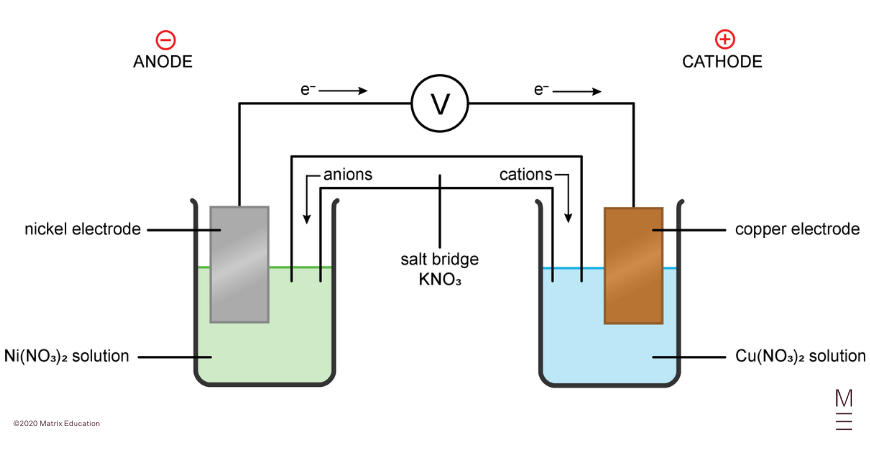The two metals (the nickel and copper) form the two electrodes – the anode is negatively charged, and the cathode is positively charged.

• Oxidation occurs at the negatively charged anode.
• Reduction occurs at the positively charged cathode.
• The overall REDOX reaction refers to the reduction and oxidation processes together.

You will need to write chemical equations for the oxidation, reduction, and overall REDOX reactions. The more reactive metal (higher in the metal activity series) will always from the anode of the galvanic cell.

In a galvanic cell, electrons flow from the anode to the cathode, and ions in the salt bridge also move as shown in the diagram to ensure the overall charge in each half of the cell remains constant.

You will need to calculate the potential of the galvanic cell (the voltage), which you can do by referring to the table of standard reduction potentials provided in exams.  The table provided by NESA is included below.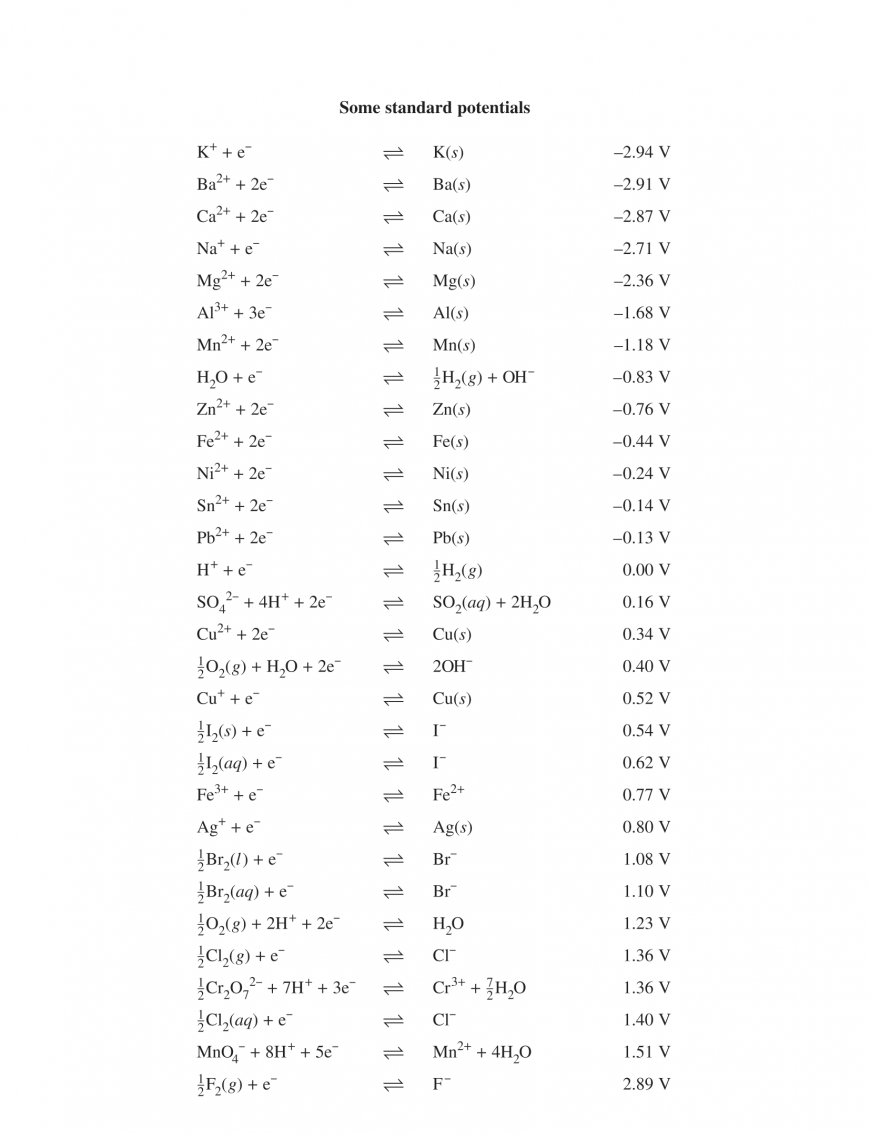You must also be able to describe changes that occur as the REDOX reaction proceeds, such as changes to the mass of the electrodes, or to the concentration of the salts (and colour of the solutions if the salts are coloured). Make sure to link what you describe with chemical equations to physical observations!

## Rates of Chemical Reaction

So far you have been developing your knowledge about different types of chemical reactions. However, the rate of a chemical reaction – how fast it proceeds, is also very important in chemistry. This will depend on a number of things, which we will discuss below.

### Collision Theory and Activation Energy

We know that a chemical reaction involves the breaking of chemical bonds, and the formation of new chemical bonds.

It makes sense that in order for this to happen…

Molecules have to be close together – they have to collide.

The following illustration shows the collision of two chlorine monoxide molecules to produce chlorine gas and oxygen gas.

In this model, the filled red circle illustrates a chlorine atom and the unfilled red circle illustrates an oxygen atom.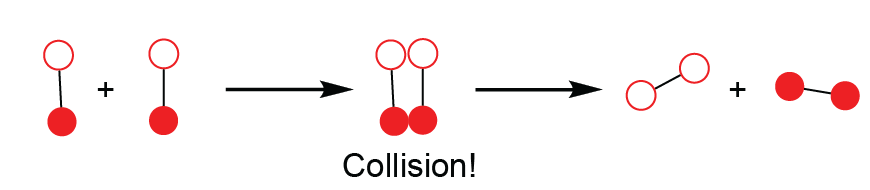For a reaction to proceed, an effective (successful) collision must occur.

In order for a collision to be effective:

• The reactant molecules must collide in the correct orientation.
• The reactant molecules must collide with sufficient energy to break covalent bonds. This is called the activation energy of the reaction – EA.

The activation energy is the “energy barrier” that must be overcome before a chemical reaction can take place.

Often, you will see a reaction coordinate diagram used to show this information, as shown below: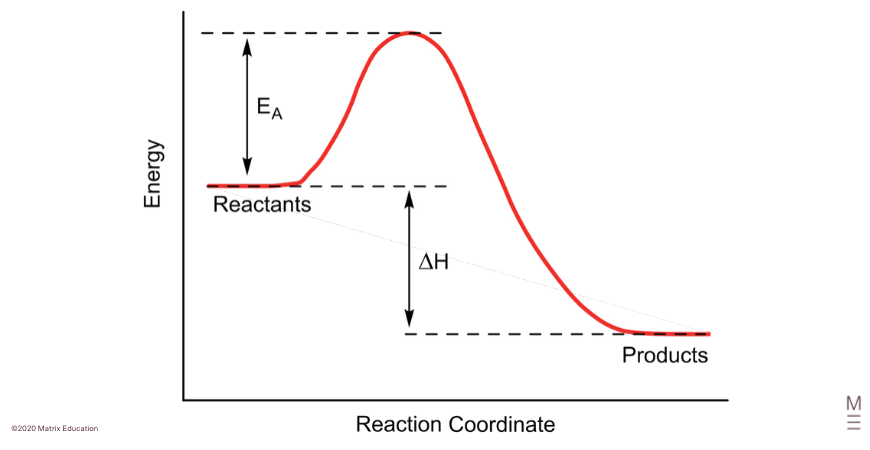The “reaction coordinate” refers to the process of the collision – reactants on the left and products on the right.

The energy difference between the reactants and products is the energy gained or released by the reaction, referred to as the enthalpy of reaction, and is given the symbol $$\Delta H$$.

The difference in energy between the reactants and the highest energy point of the curve is the activation energy. This is the energy that is required for the reaction to proceed.

You can think about this like rolling a ball over a hill – you need to push the ball to the top, but once you are over the top of the hill, it is downhill to the products!

### Factors Affecting Rates of Reaction

The rate of a chemical reaction is the speed at which a chemical reaction proceeds, and is often measured by the change in concentration of a product or reactant over time.

A number of factors affect the rate of a chemical reaction. These are discussed below, but each can be related back to collision theory.

Importantly, the rate of a chemical reaction is proportional to the frequency of effective collisions.

The frequency of effective collisions is dependent upon the four following factors:

1. Temperature­

2. Surface Area of Reactants

3. Concentration of Reactants

4. Catalysts

increasing the temperature, surface area and concentration of reactants, and adding a catalyst will result in a larger number of successful collisions per second, and will hence increase the rate of reaction.

© Matrix Education and www.matrix.edu.au, 2022. Unauthorised use and/or duplication of this material without express and written permission from this site’s author and/or owner is strictly prohibited. Excerpts and links may be used, provided that full and clear credit is given to Matrix Education and www.matrix.edu.au with appropriate and specific direction to the original content.